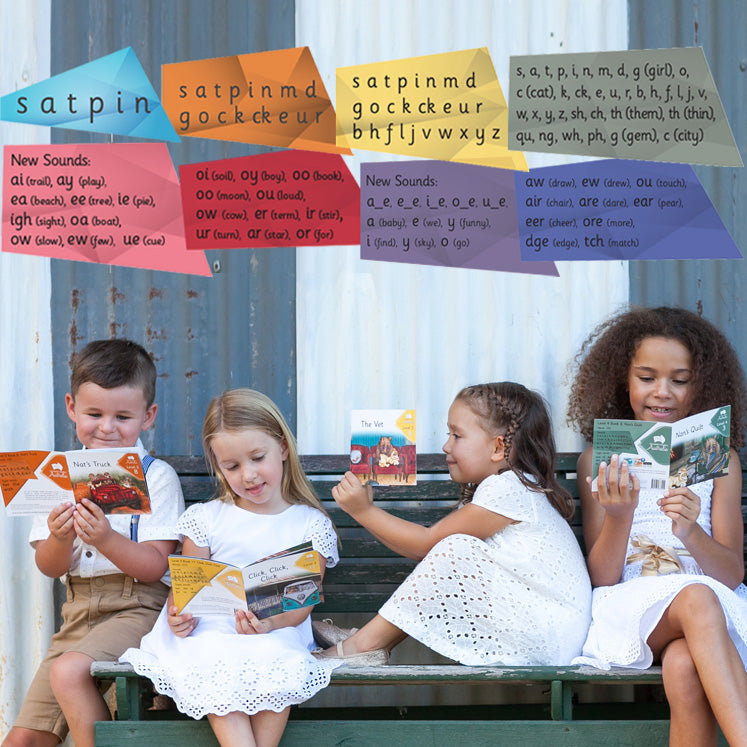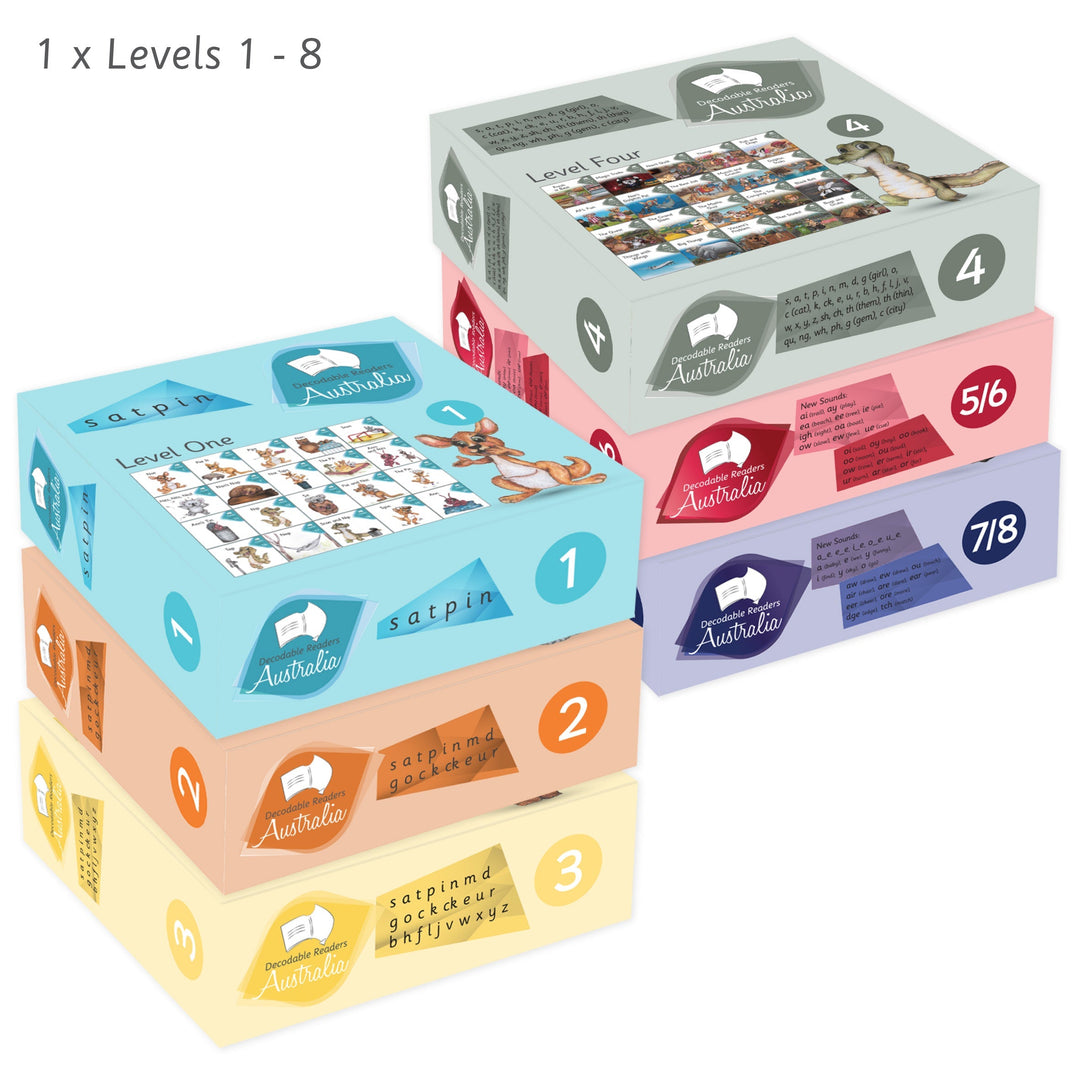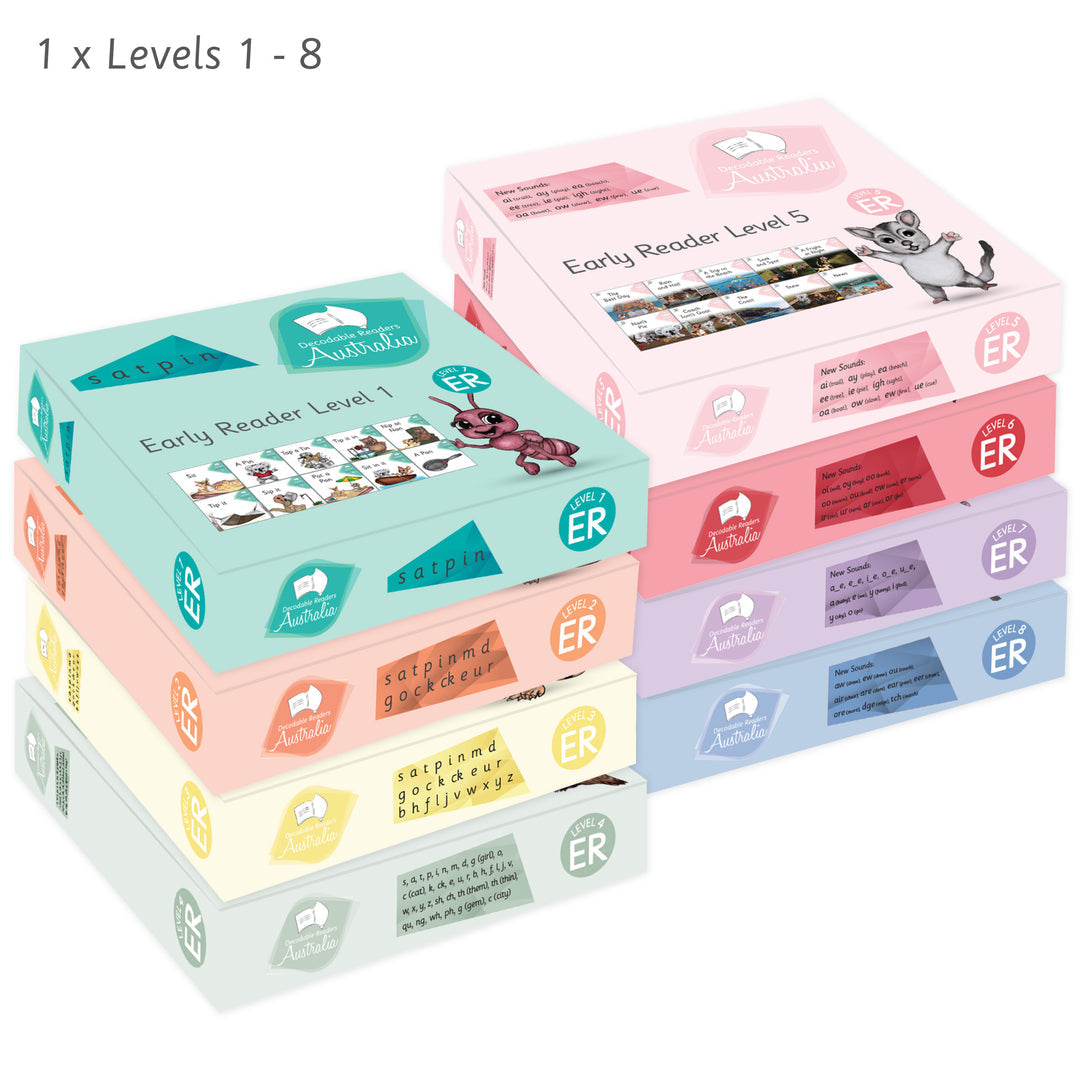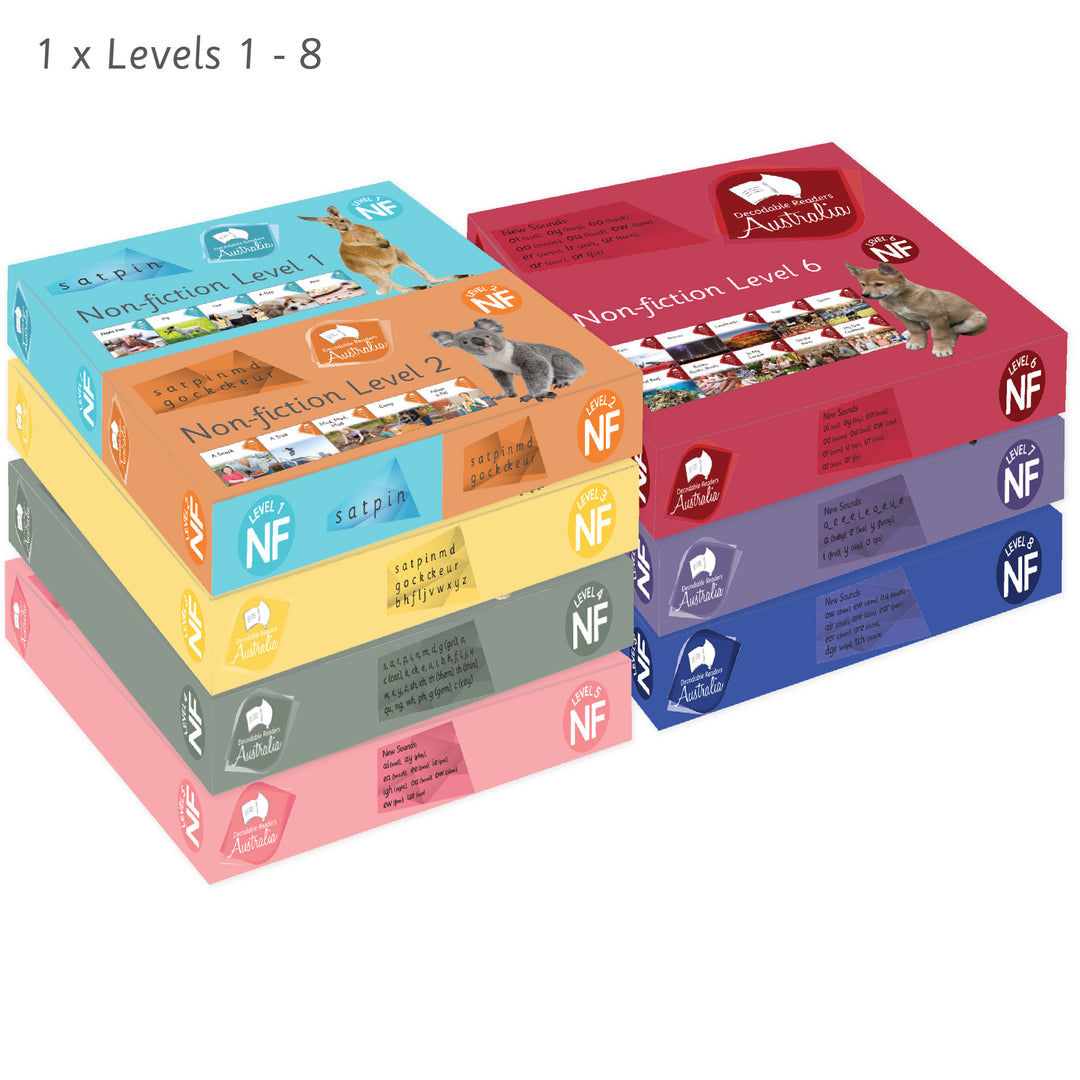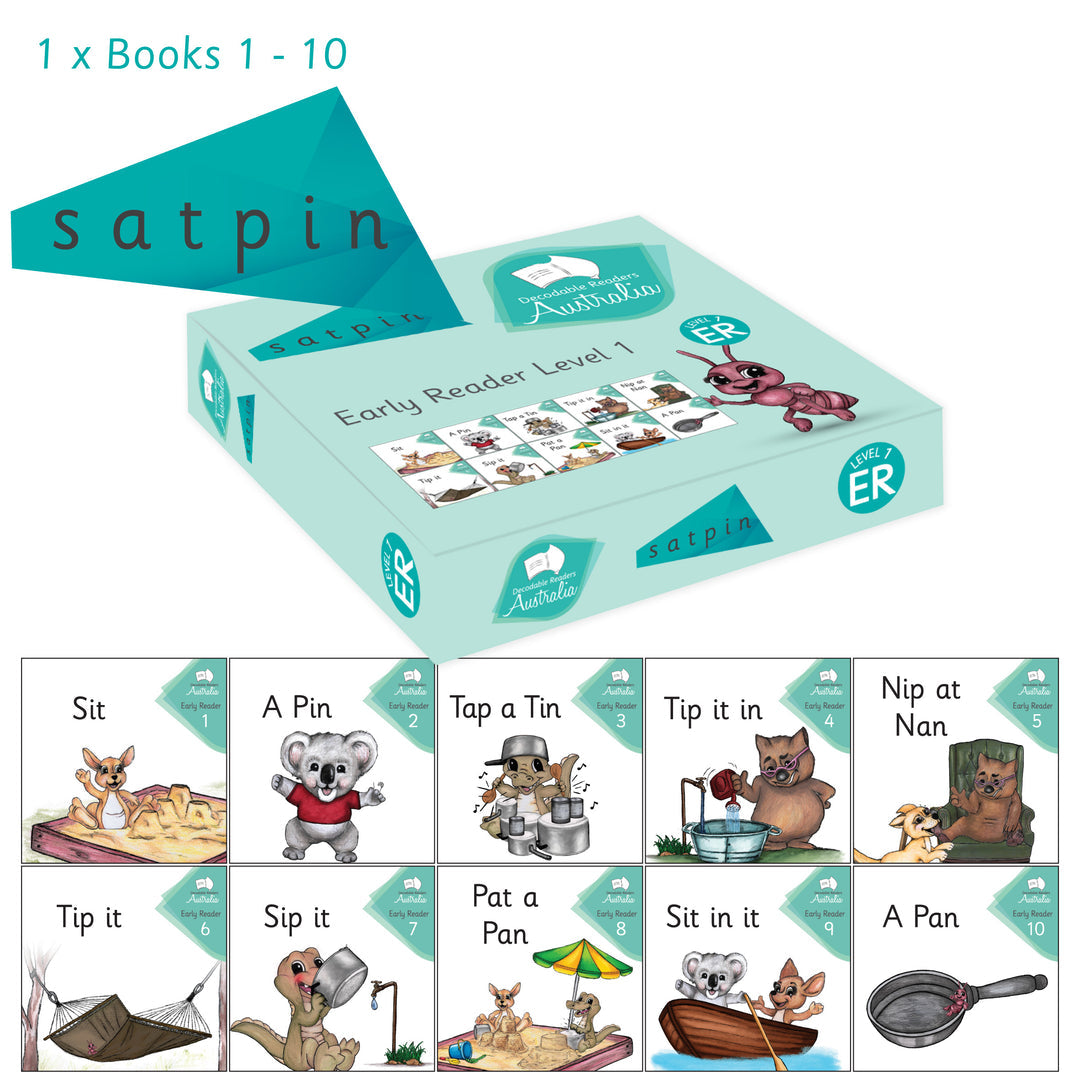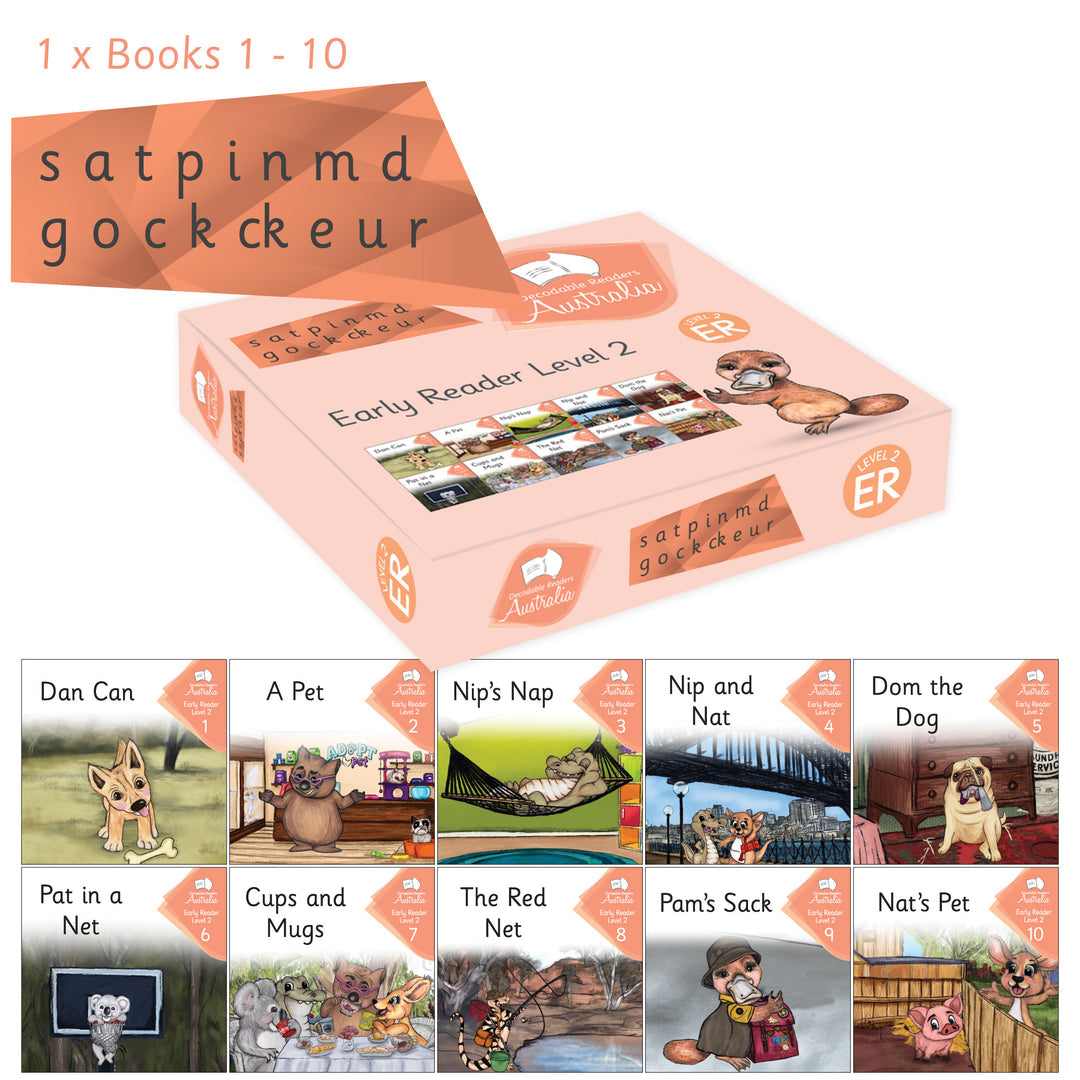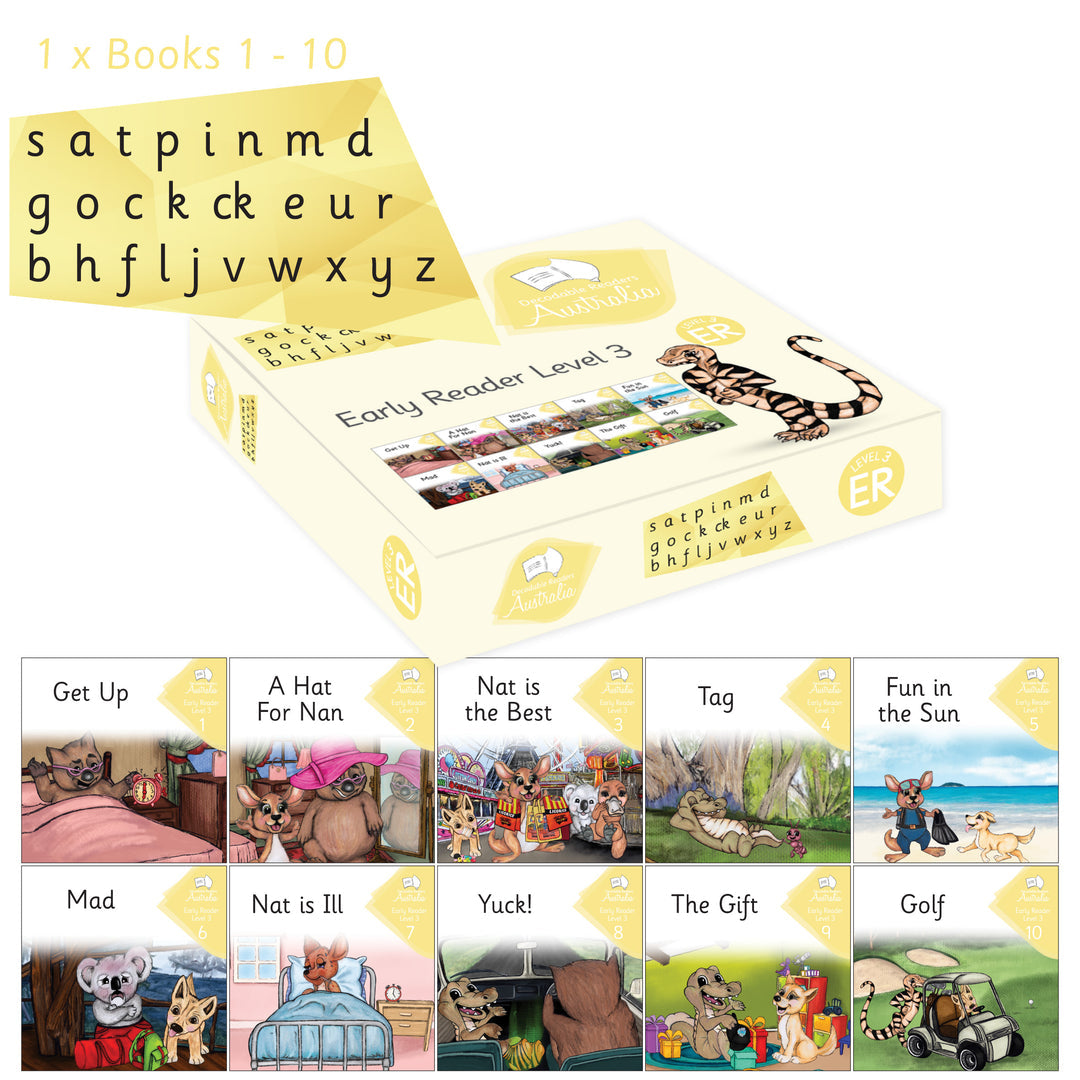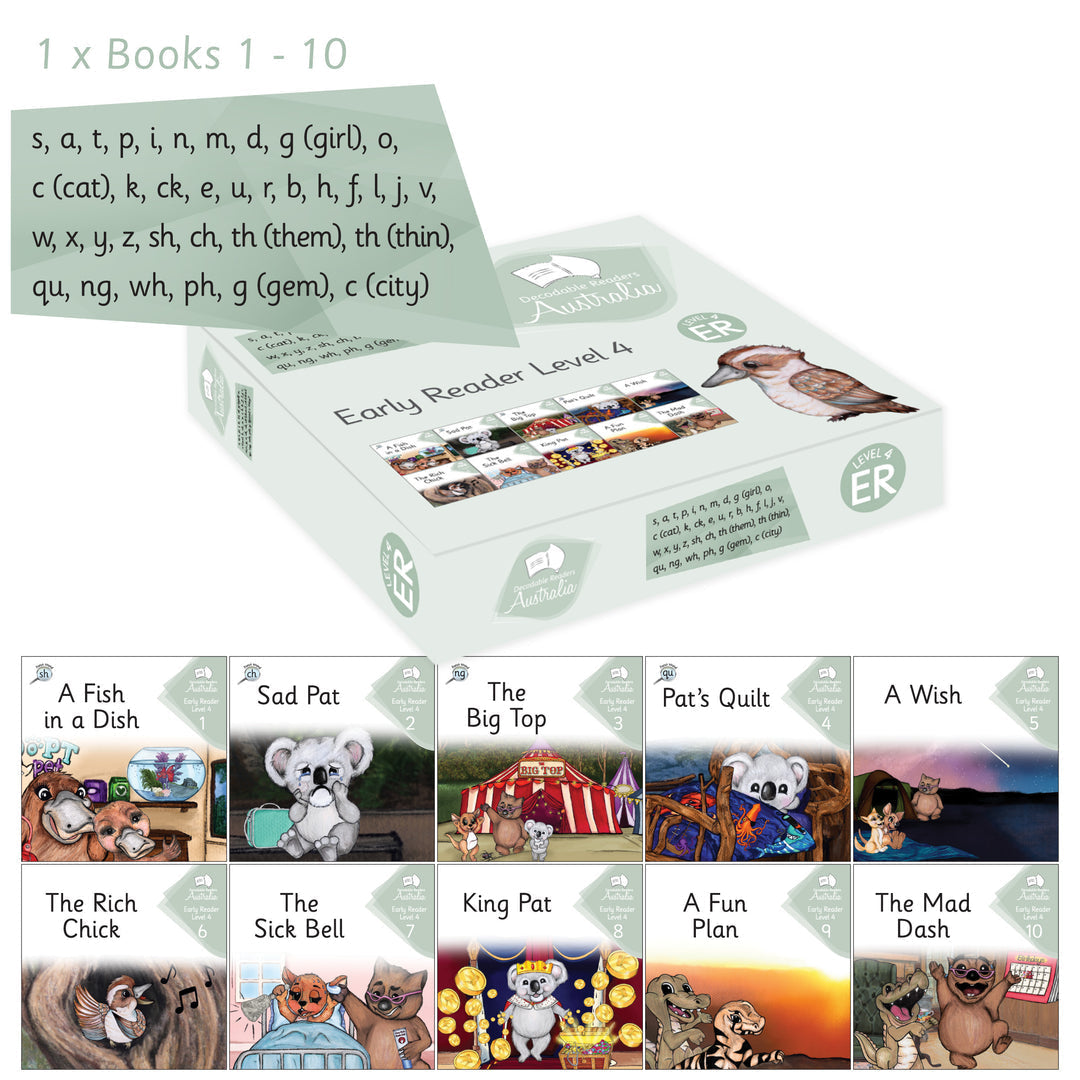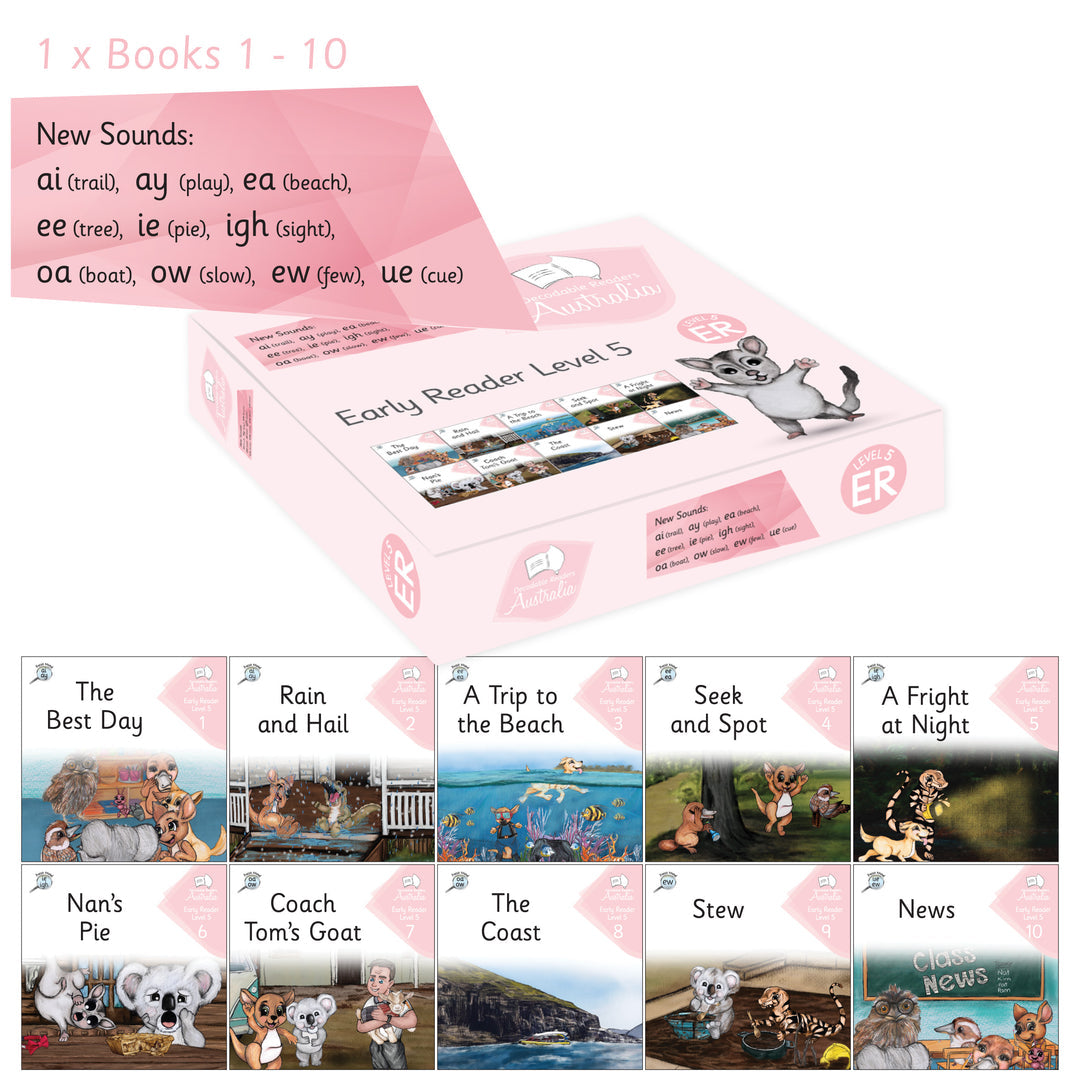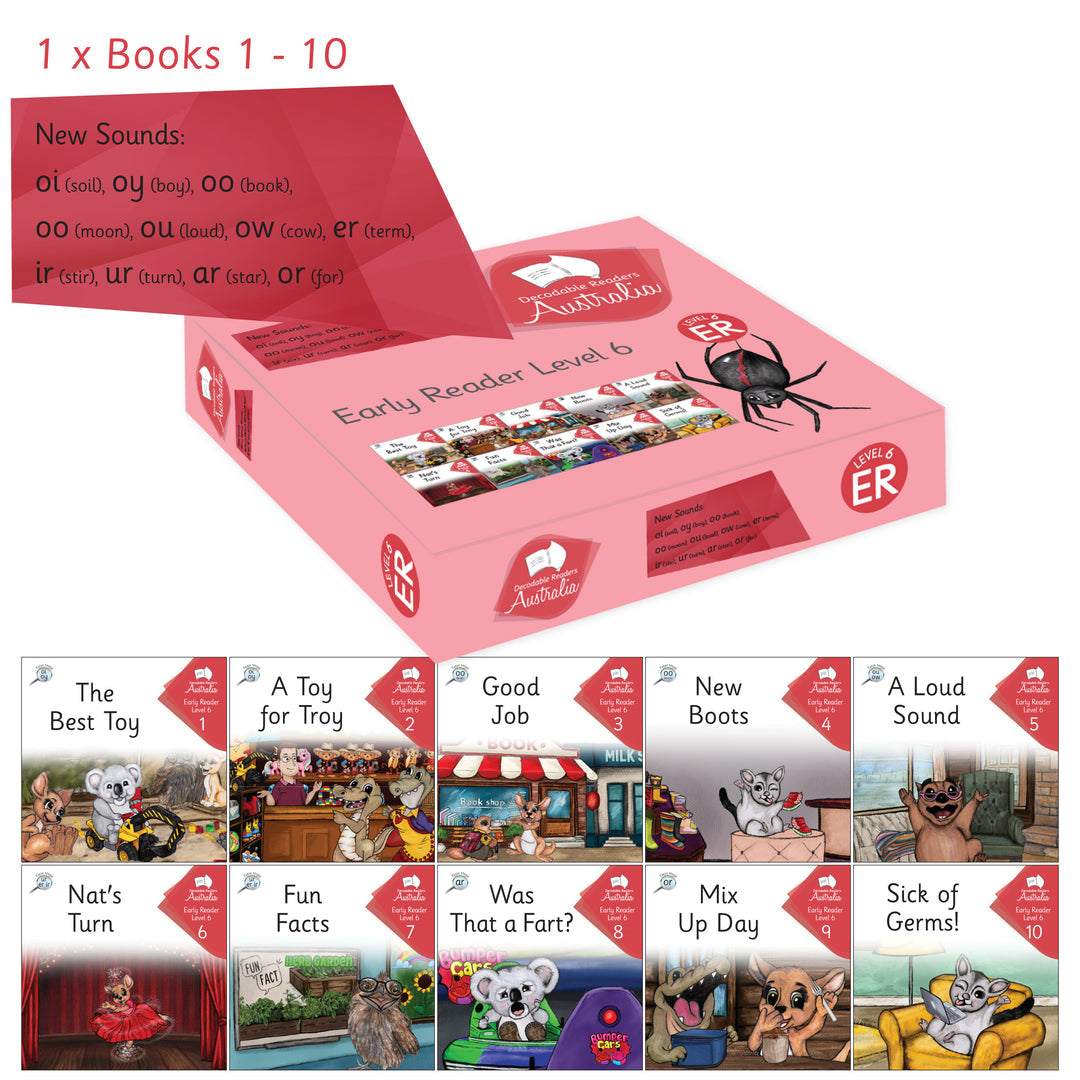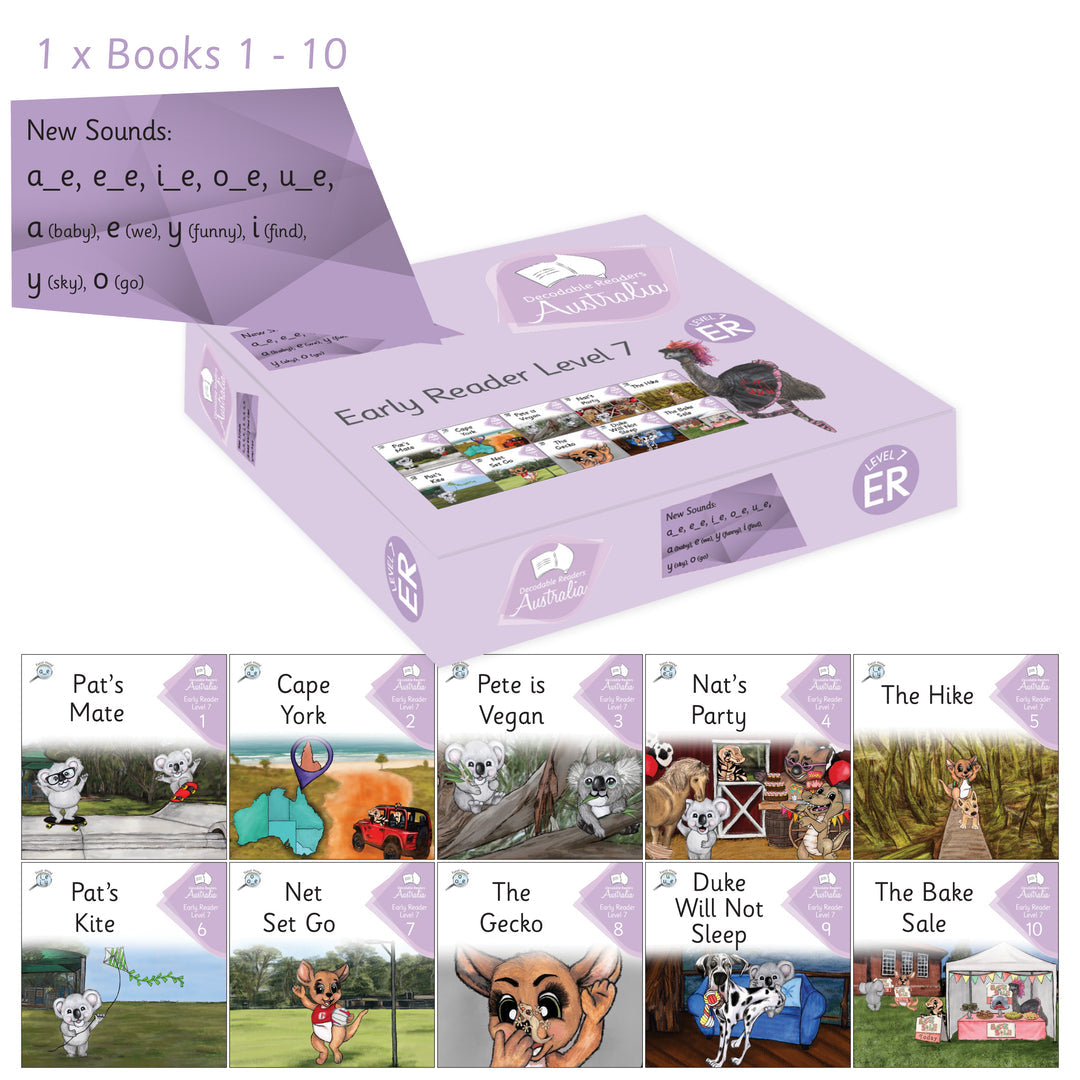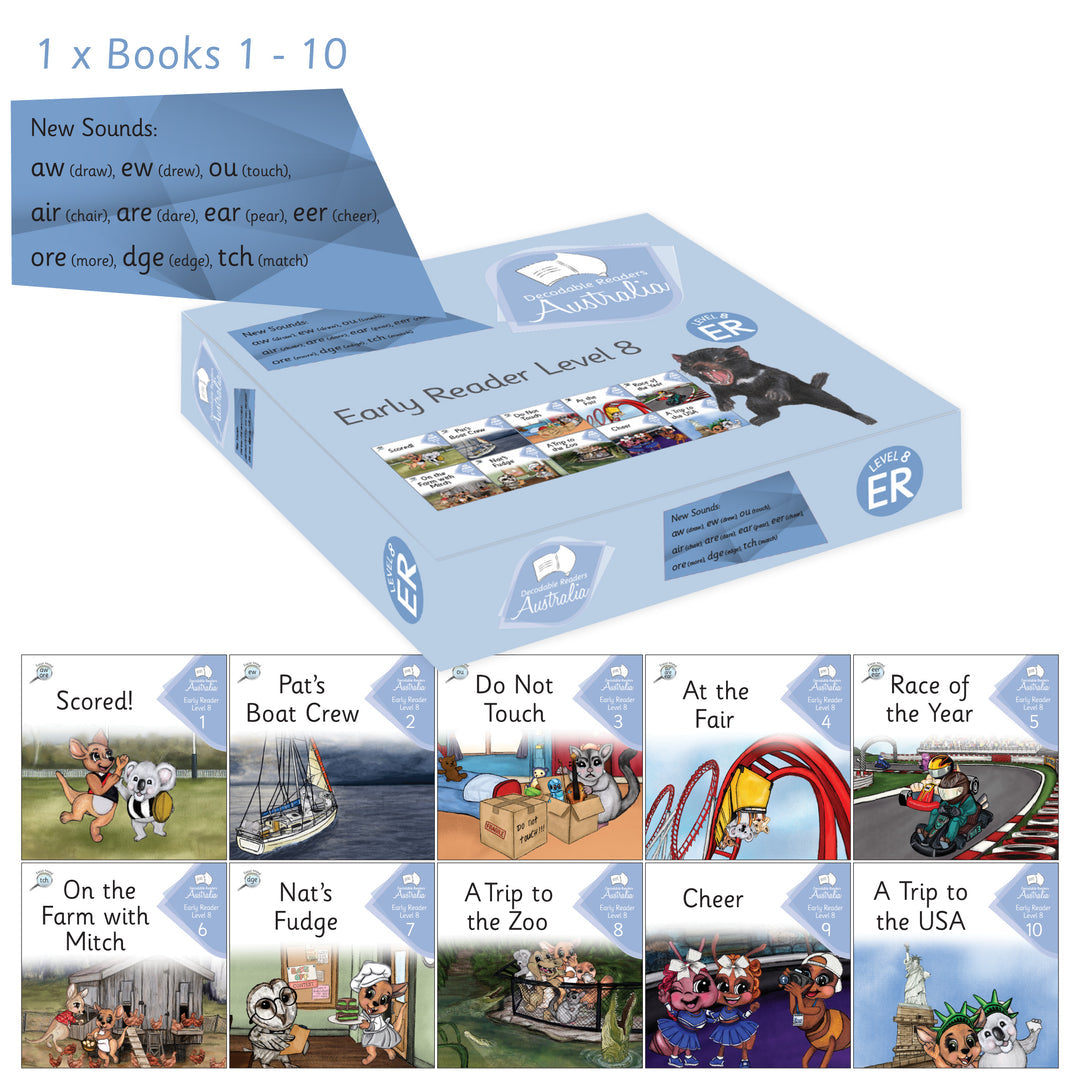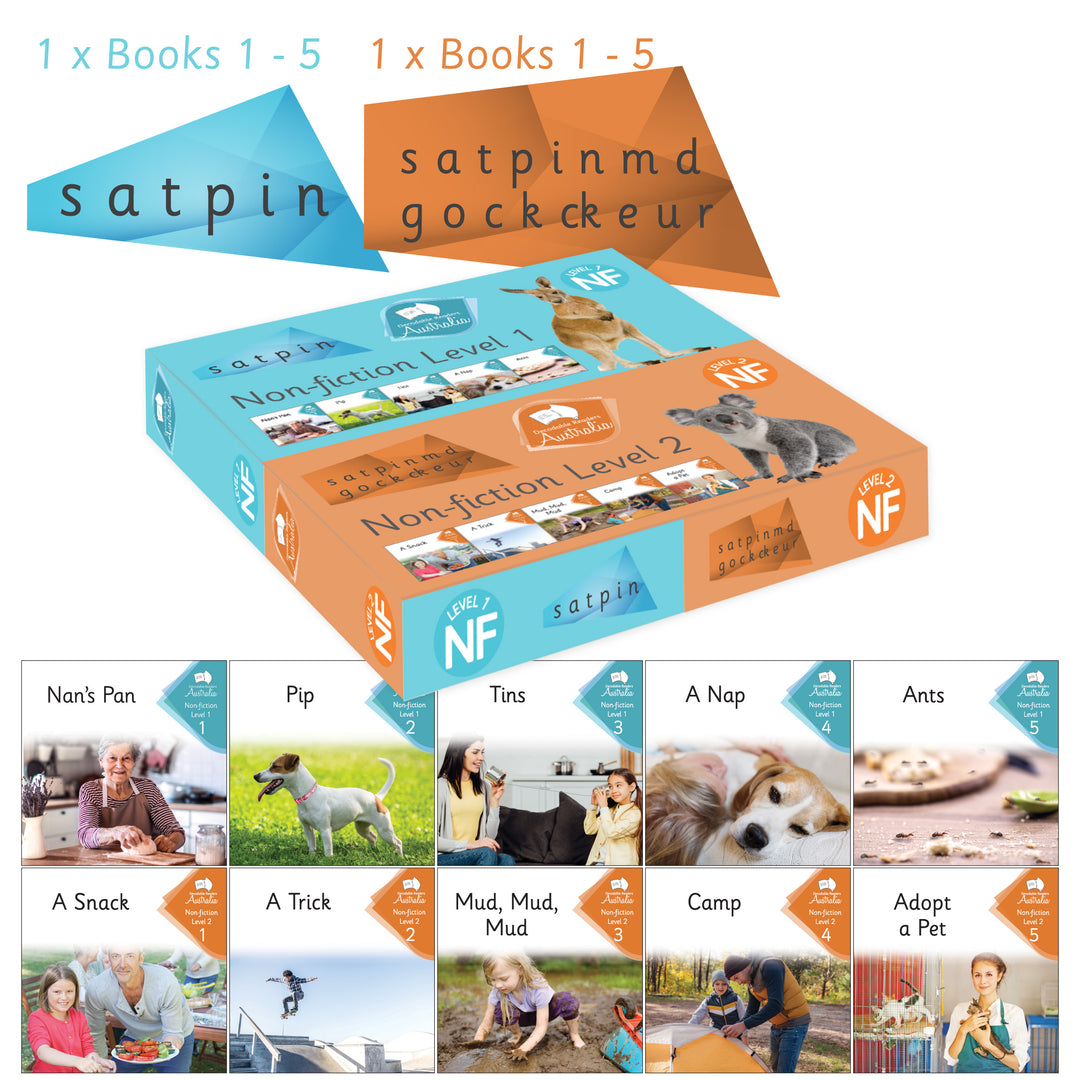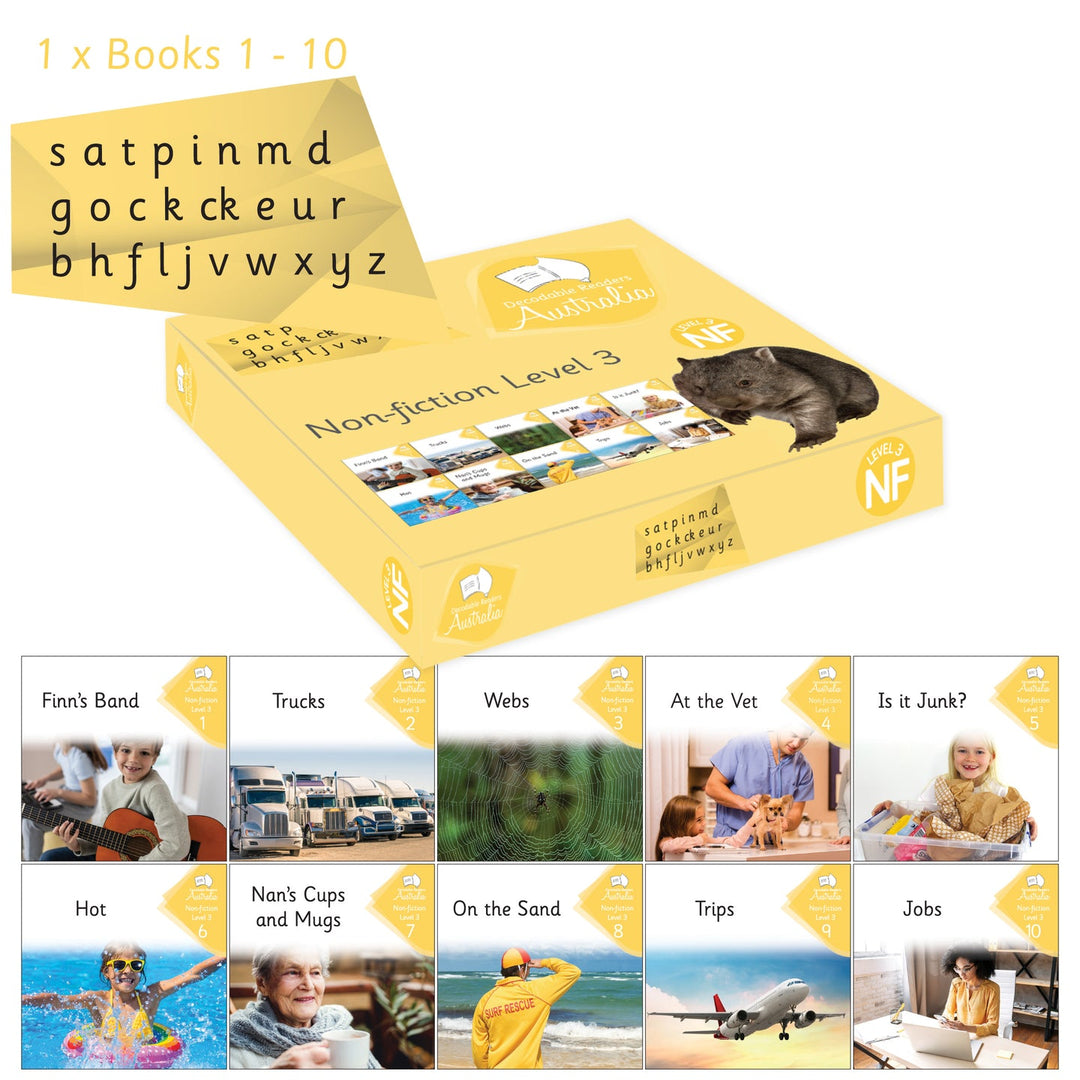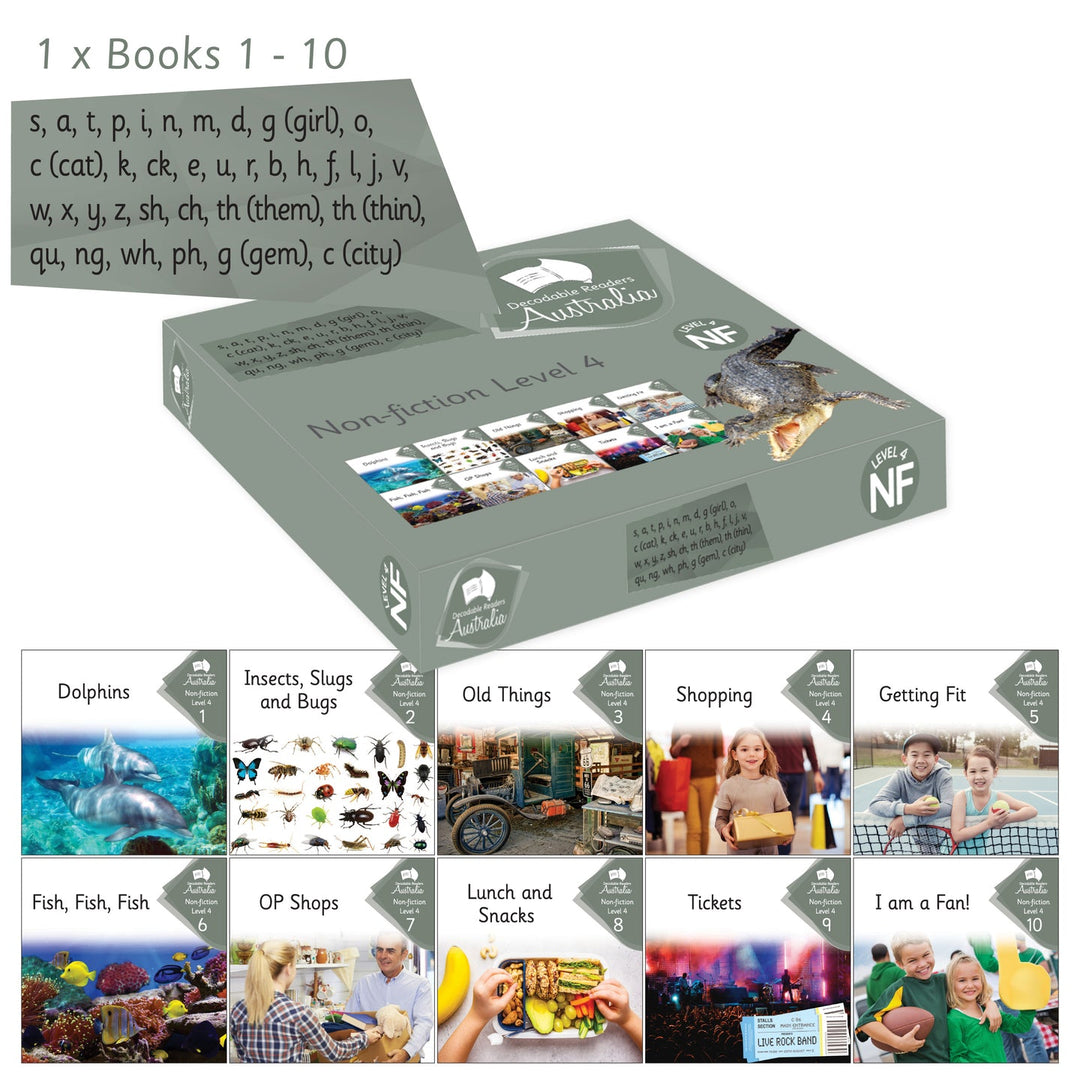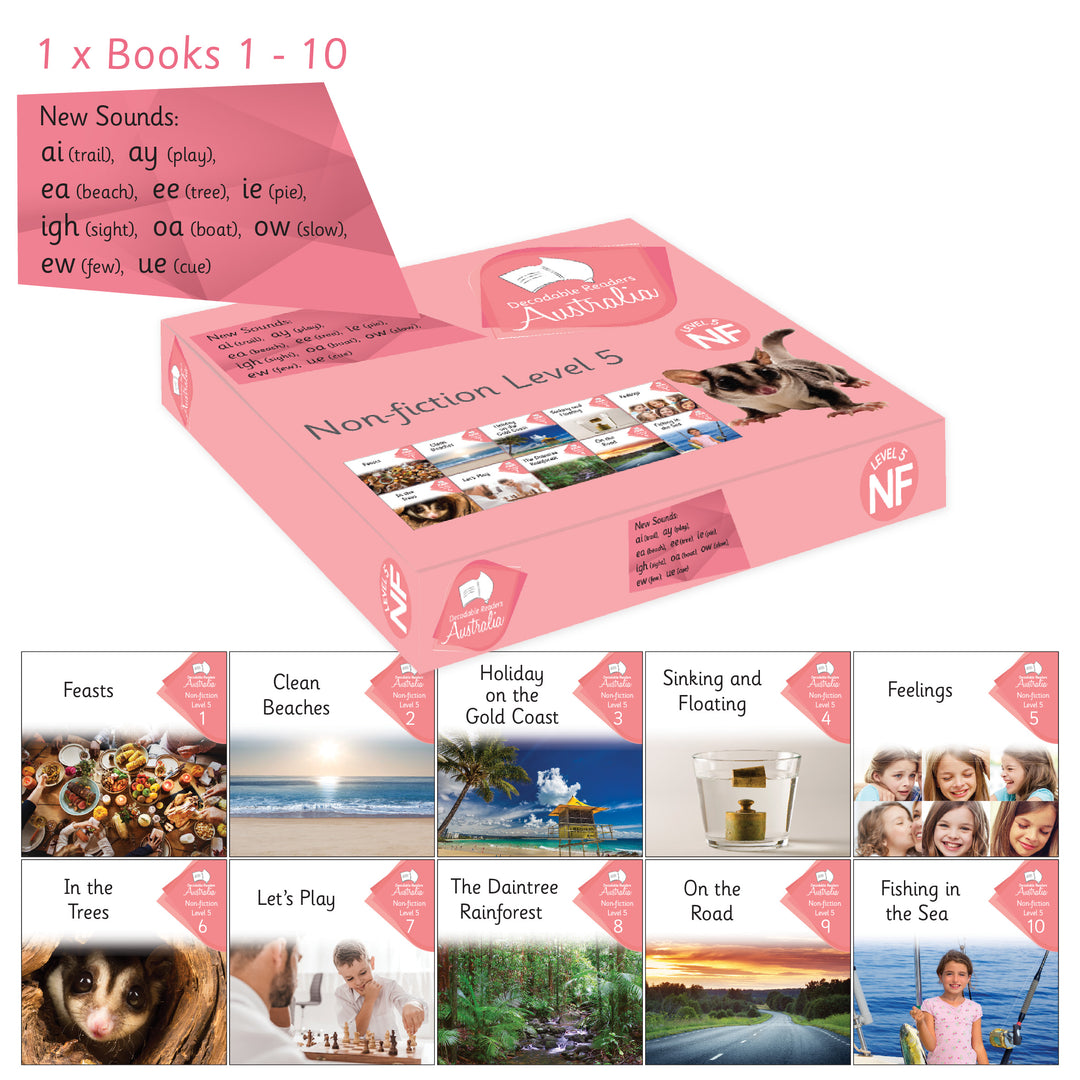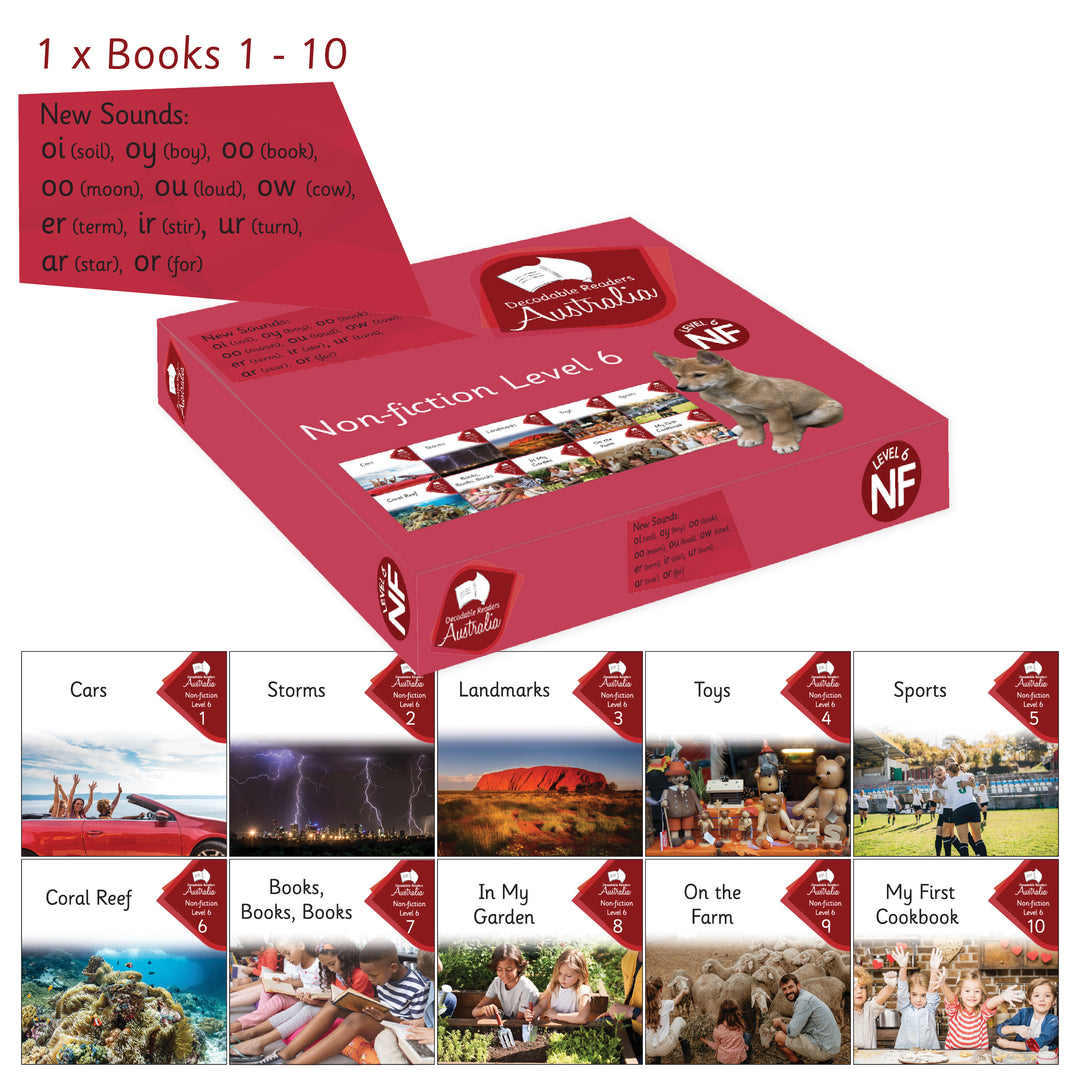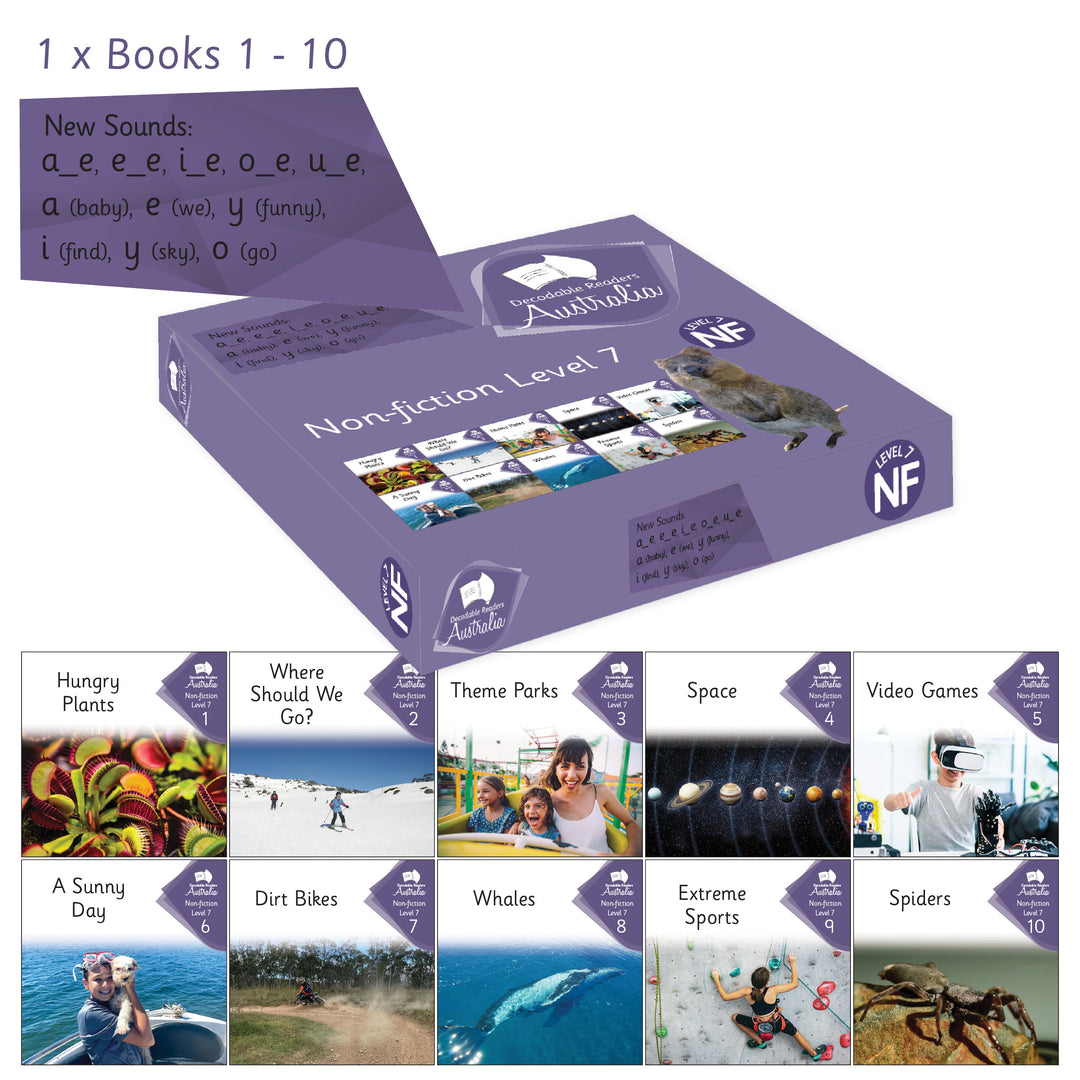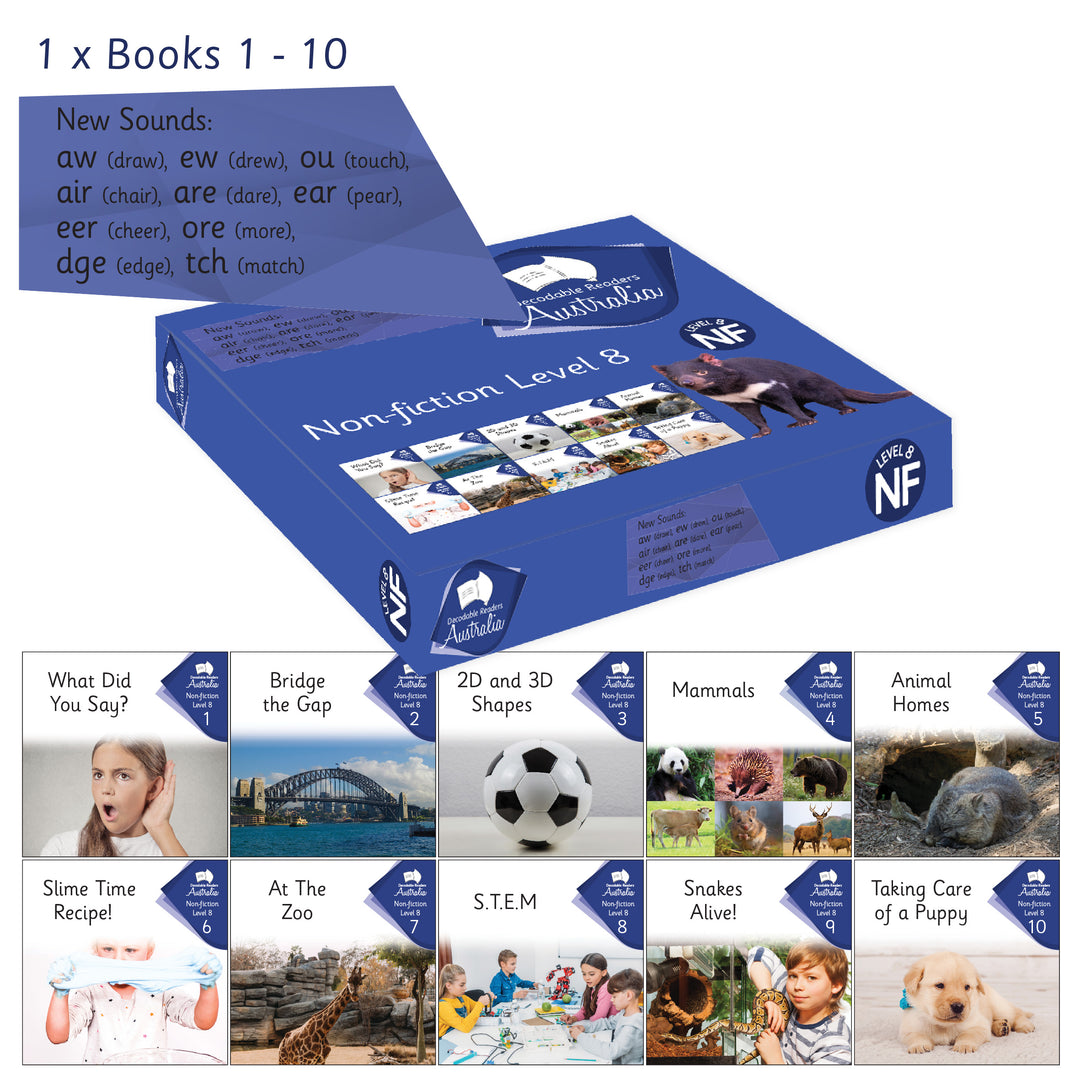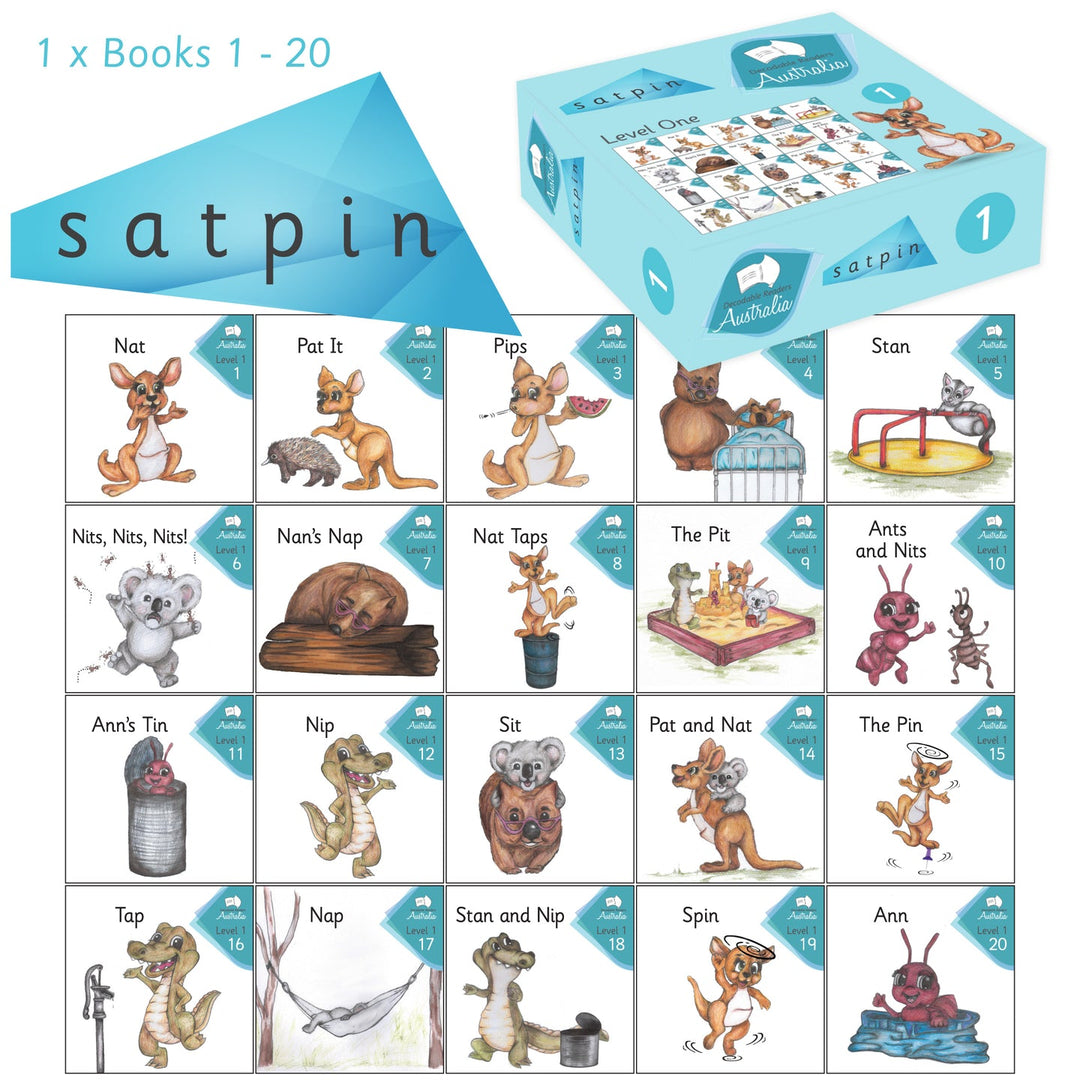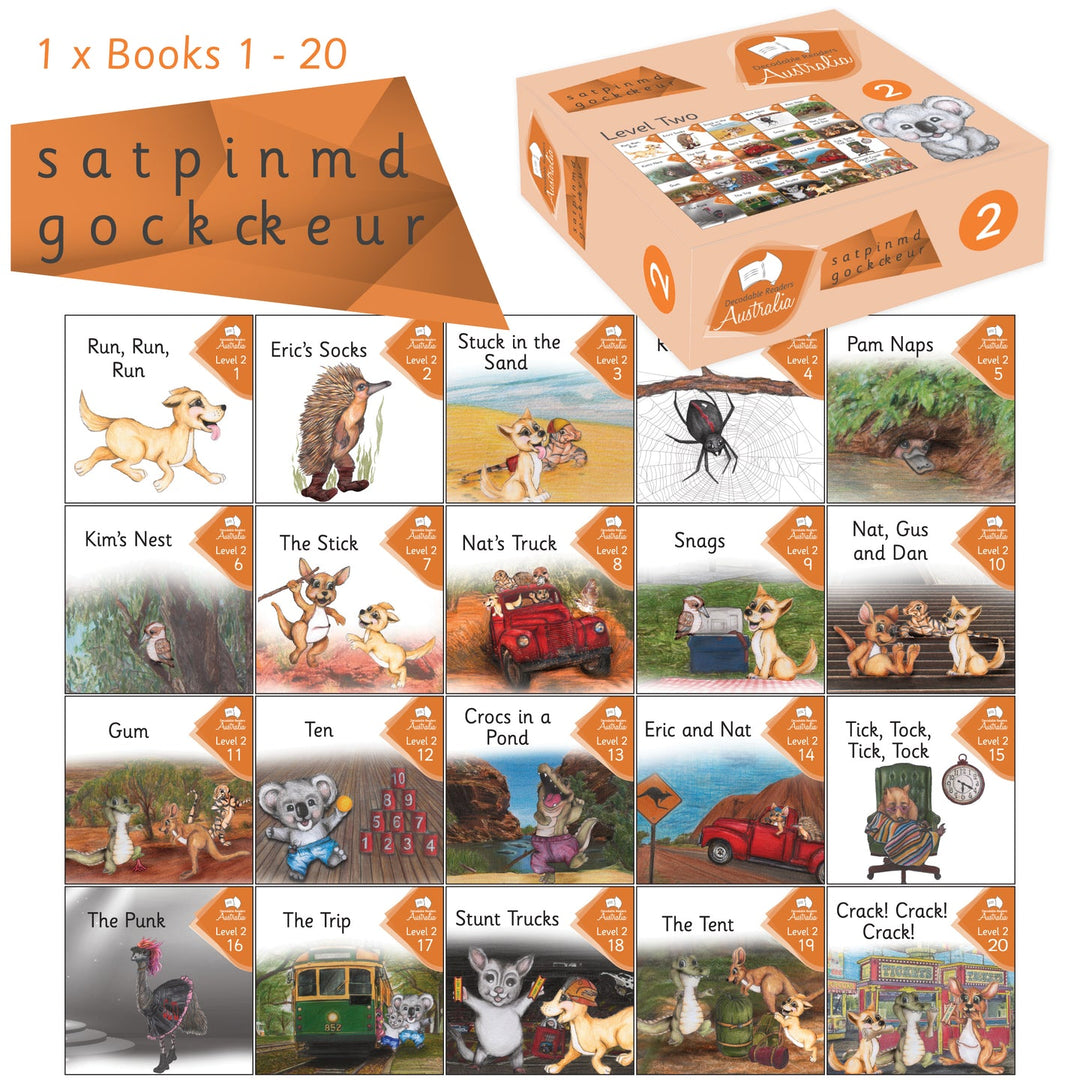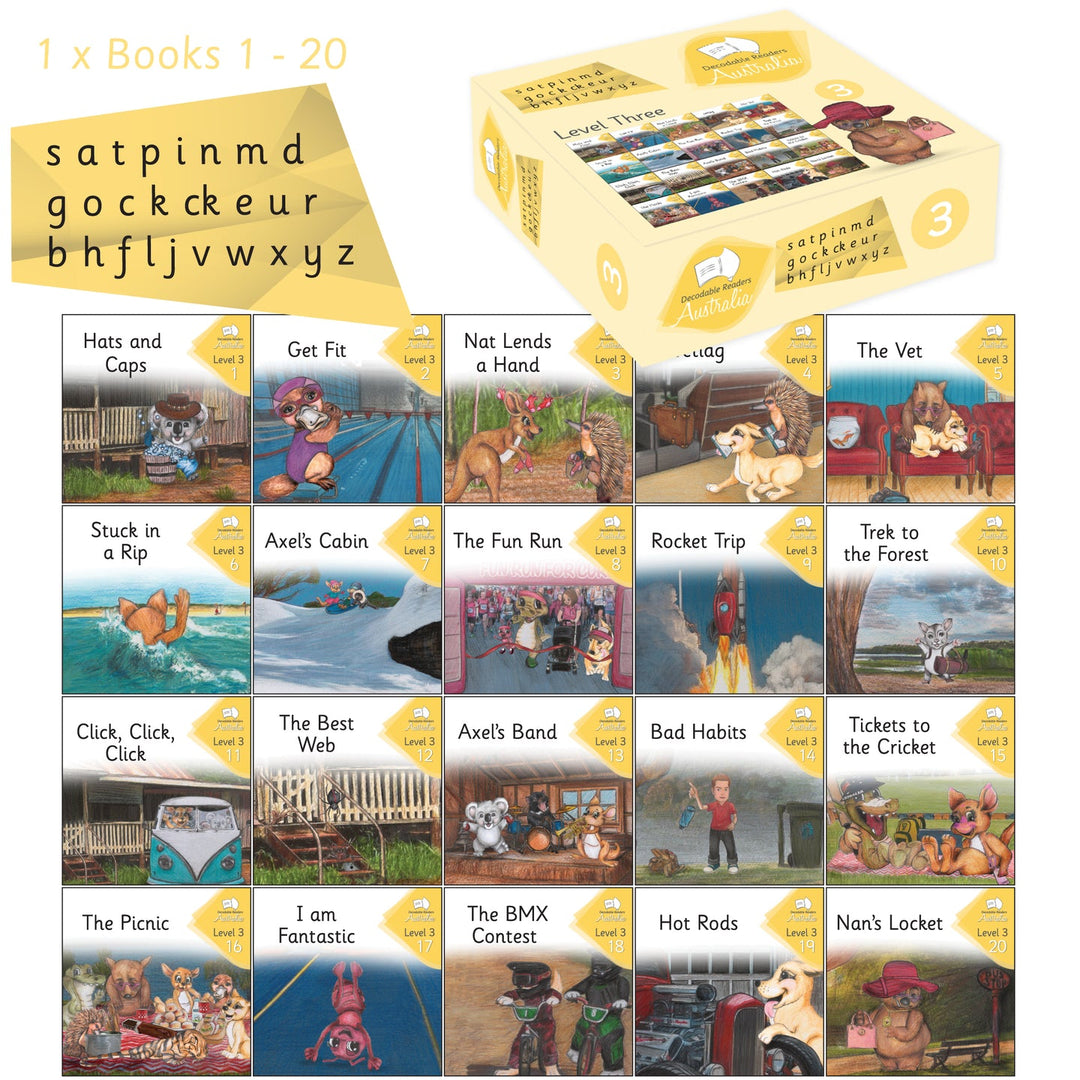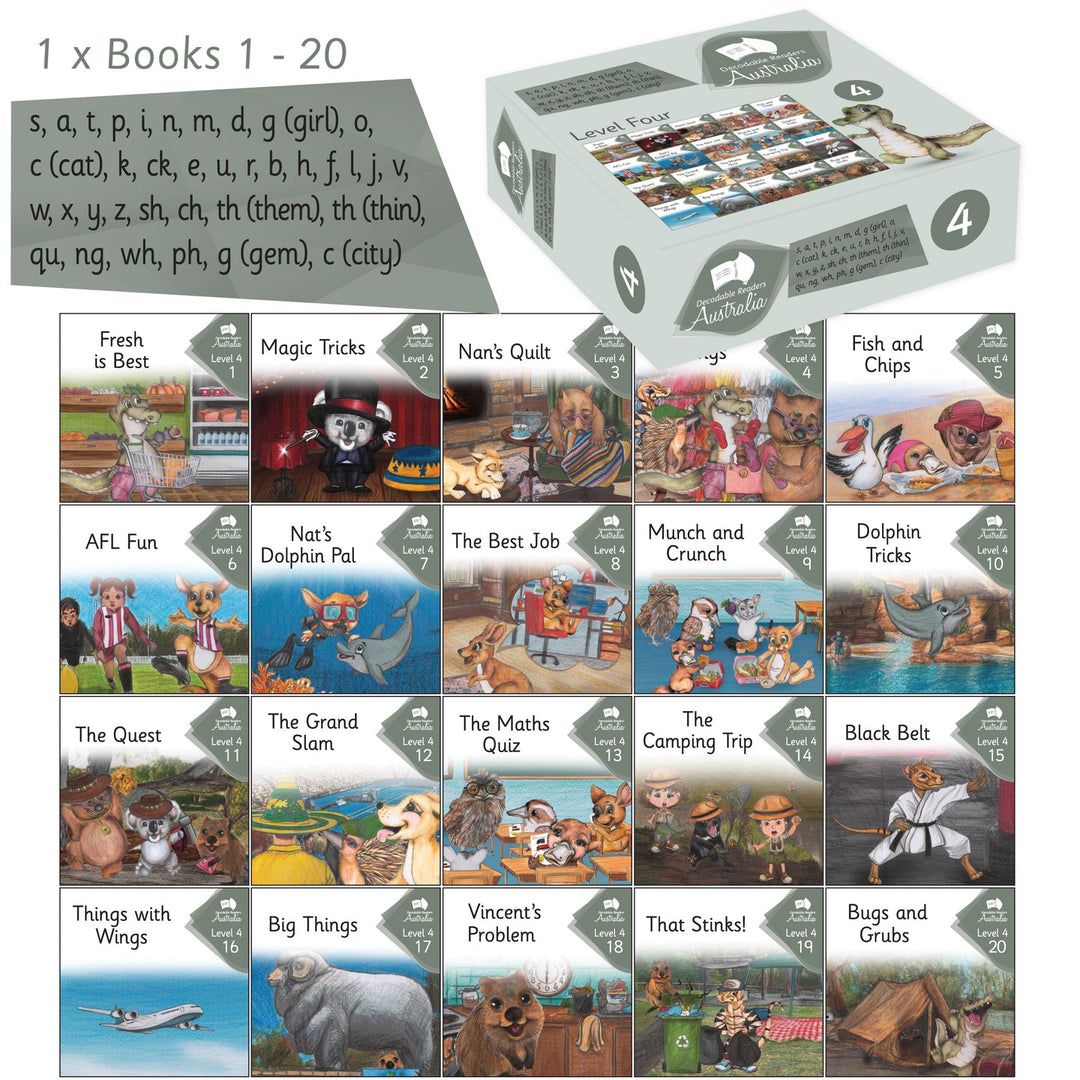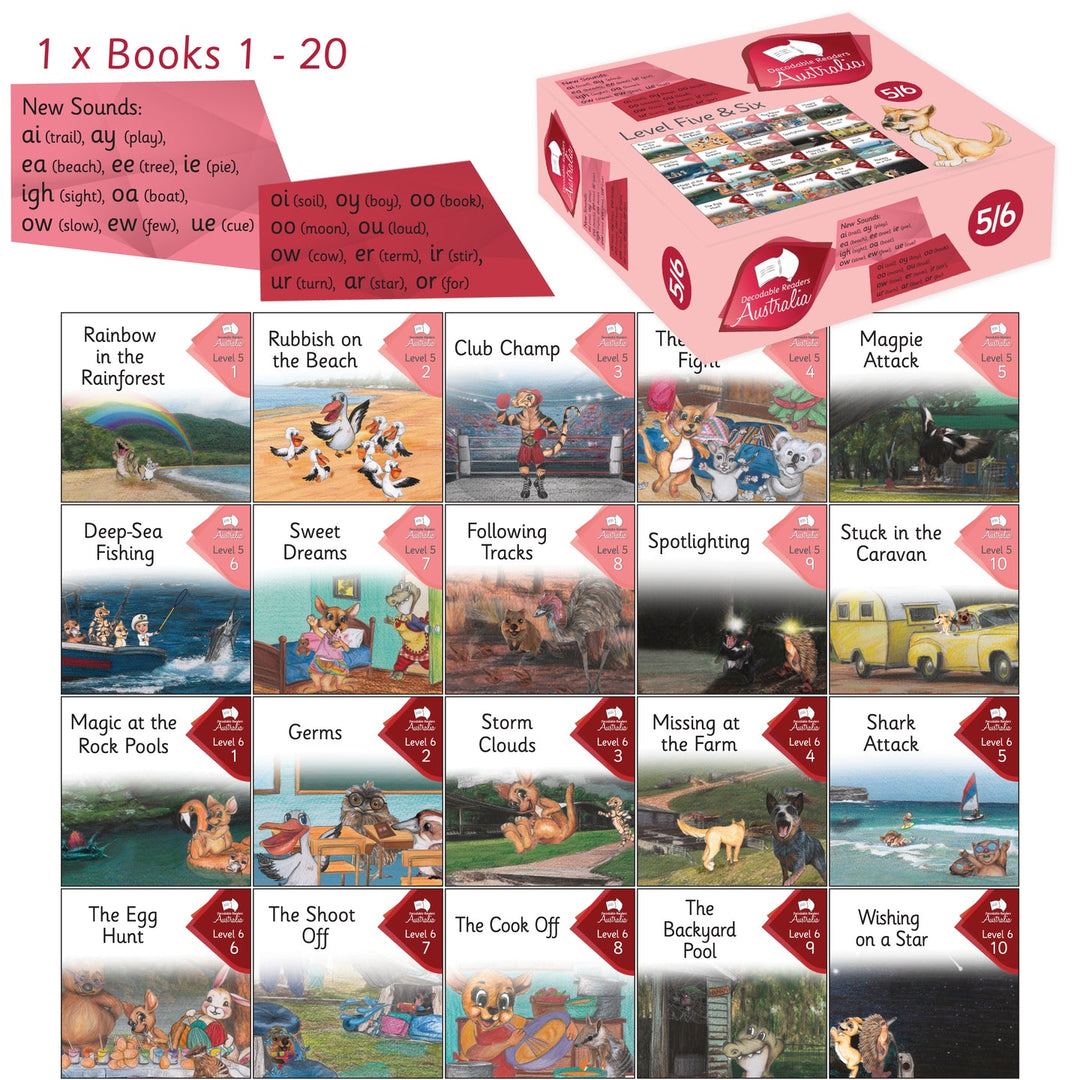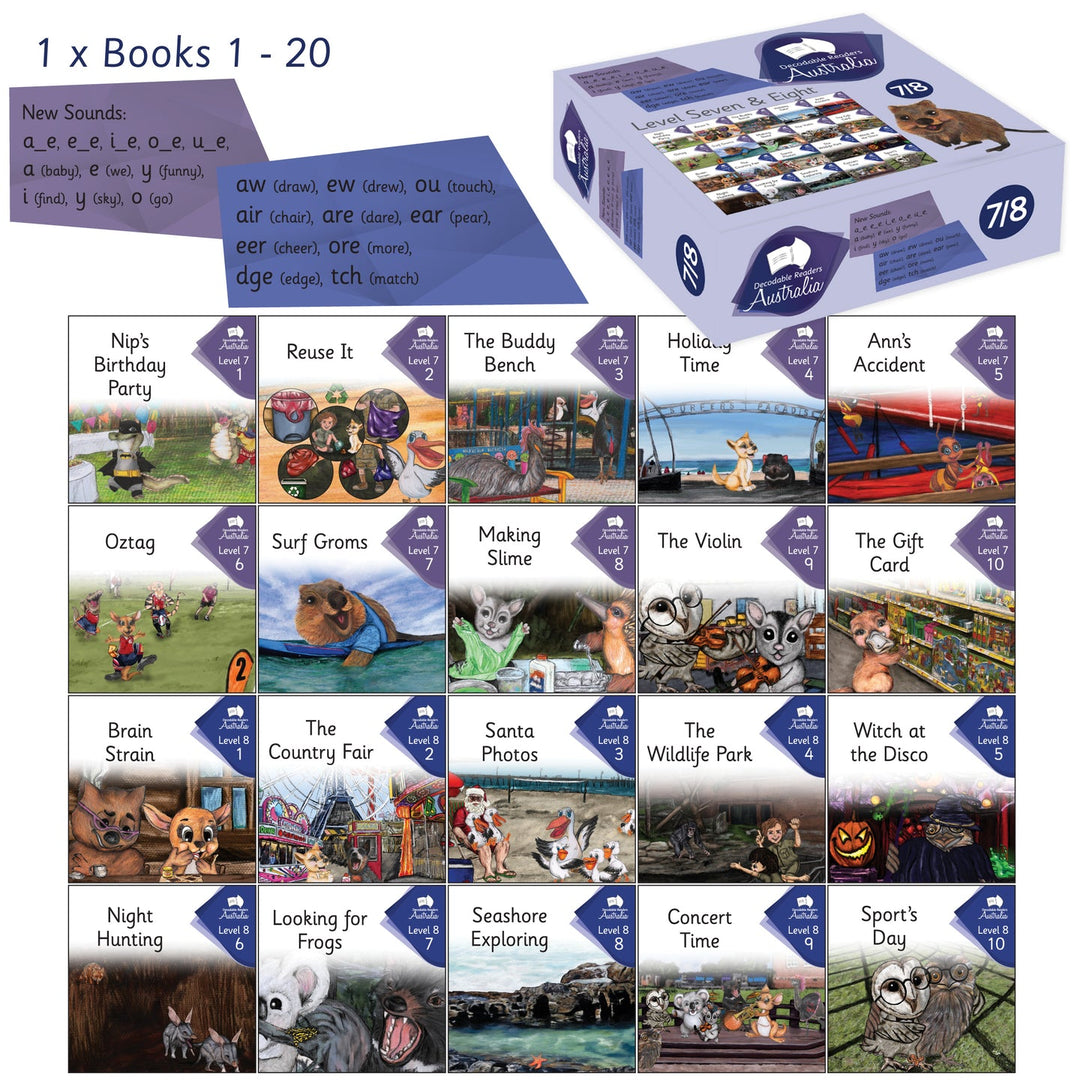# Combined Complete INDIVIDUAL Set - Levels 1 - 8 (Early Readers + Nonfiction + Main Fiction Series)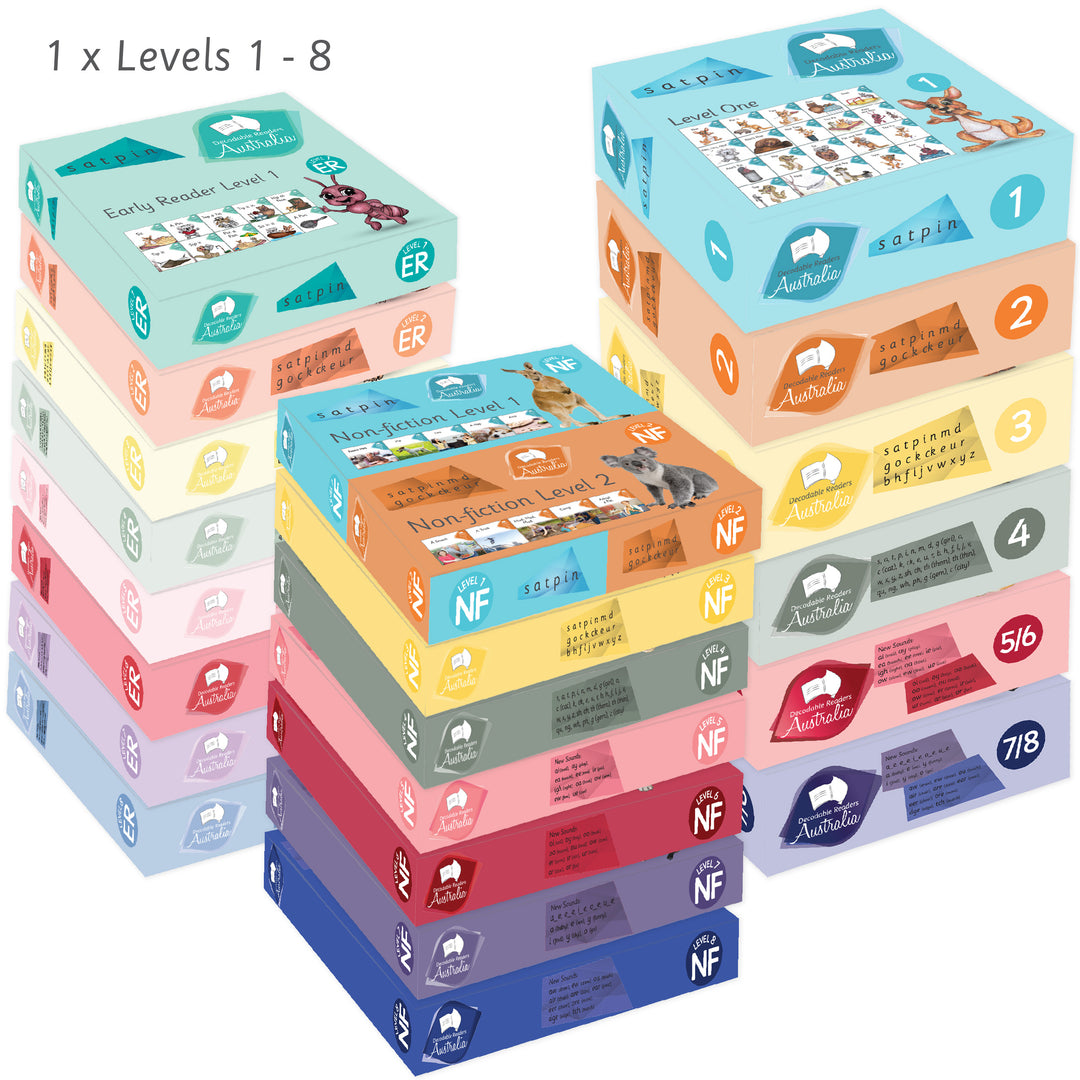\$1,500.00 AUD

\$1,782.00 AUD

COMBINED COMPLETE SETS LEVEL 1-8- INDIVIDUAL SETS (Early Readers + Nonfiction + Main Fiction Series)

Levels 1 to 8 , 1 copy of each Level 1 – 8 title

Level 1 = 10 Books
Level 2 = 10 Books
Level 3 = 10 Books
Level 4 = 10 Books
Level 5 = 10 Books
Level 6 = 10 Books
Level 7 = 10 Books
Level 8 = 10 Books

= 80 Books

Nonfiction:

Level 1/2 = 10 Books
Level 3 = 10 Books
Level 4 = 10 Books
Level 5 = 10 Books
Level 6 = 10 Books
Level 7 = 10 Books
Level 8 = 10 Books

= 70 Books

Main Fiction Series

Level 1 = 20 Books
Level 2 = 20 Books
Level 3 = 20 Books
Level 4 = 20 Books
Level 5/6 = 20 Books
Level 7/8 = 20 Books
= 120 Books

270 books in total

These decodable texts allow children time to consolidate their phonetic code knowledge before introducing more of the English written code.

Sight word lists for each series can be downloaded on our Free Resources page

All nonfiction books contain pre reading discussion points to build knowledge and post-reading comprehension questions to also check for understanding.